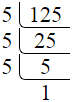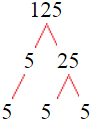# Factors of 125The factors of 125 are 1, 5, 25, and 125 i.e. F125 = {1, 5, 25, 125}. The factors of 125 are all the numbers that can divide 125 without leaving a remainder.

We can check if these numbers are factors of 125 by dividing 125 by each of them. If the result is a whole number, then the number is a factor of 125. Let's do this for each of the numbers listed above:

·        1 is a factor of 125 because 125 divided by 1 is 125.

·        5 is a factor of 125 because 125 divided by 5 is 25.

·        25 is a factor of 125 because 125 divided by 25 is 5.

·        125 is a factor of 125 because 125 divided by 125 is 1.

## How to Find Factors of 125?

1 and the number itself are the factors of every number. So, 1 and 125 are two factors of 125. To find the other factors of 125, we can start by dividing 125 by the numbers between 1 and 125. If we divide 125 by 2, we get a remainder of 1. Therefore, 2 is not a factor of 125. If we divide 125 by 3, we get a remainder of 2. Therefore, 3 is not a factor of 125.

Next, we can check if 4 is a factor of 125. If we divide 125 by 4, we get a remainder of 1. Therefore, 4 is not a factor of 125. If we divide 125 by 5, we get a remainder of 0. Therefore, 5 is a factor of 125. We can continue this process for all the possible factors of 125.

Through this process, we can find that the factors of 125 are 1, 5, 25, and 125. These are the only numbers that can divide 125 without leaving a remainder.

********************

********************

## Properties of the Factors of 125

The factors of 125 have some interesting properties. One of the properties is that the sum of the factors of 125 is equal to 156. We can see this by adding all the factors of 125 together:

1 + 5 + 25 + 125 = 156

Another property of the factors of 125 is that they are all odd numbers. This is because 125 is an odd number, and any even number cannot divide an odd number without leaving a remainder.

Another property of the factors of 125 is that the only prime factor of 125 is 5.

## Applications of the Factors of 125

The factors of 125 have several applications in mathematics. One of the applications is in finding the highest common factor (HCF) of two or more numbers. The HCF is the largest factor that two or more numbers have in common. For example, to find the HCF of 125 and 25, we need to find the factors of both numbers and identify the largest factor they have in common. The factors of 125 are 1, 5, 25, and 125. The factors of 25 are 1, 5, and 25. The largest factor that they have in common is 25. Therefore, the HCF of 125 and 25 is 25.

Another application of the factors of 125 is in prime factorization. Prime factorization is the process of expressing a number as the product of its prime factors. The only prime factor of 125 is 5. We can express 125 as:

125 = 5 × 5 × 5

We can do prime factorization by division and factor tree method also. Here is the prime factorization of 125 by division method,125 = 5 × 5 × 5

Here is the prime factorization of 125 by the factor tree method,125 = 5 × 5 × 5

## Conclusion

The factors of 125 are the numbers that can divide 125 without leaving a remainder. The factors of 125 are 1, 5, 25, and 125. The factors of 125 have some interesting properties, such as being odd numbers and having a sum of 156. The factors of 125 have several applications in mathematics, such as finding the highest common factor and prime factorization.